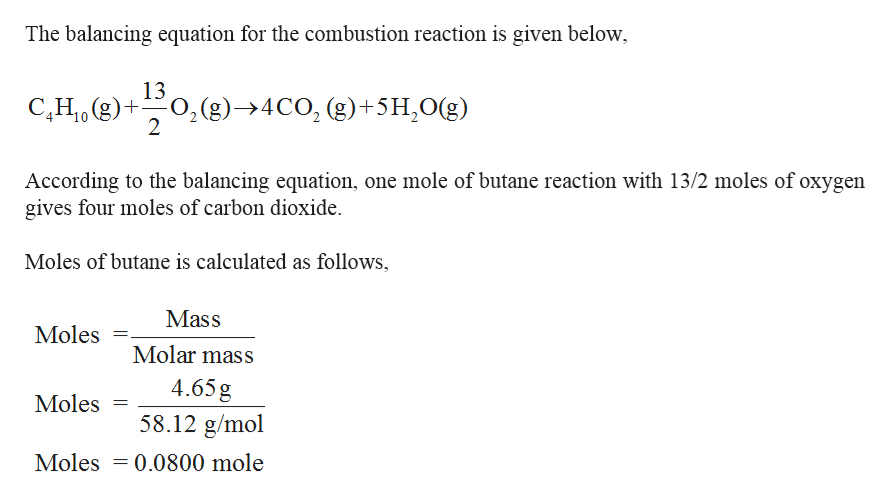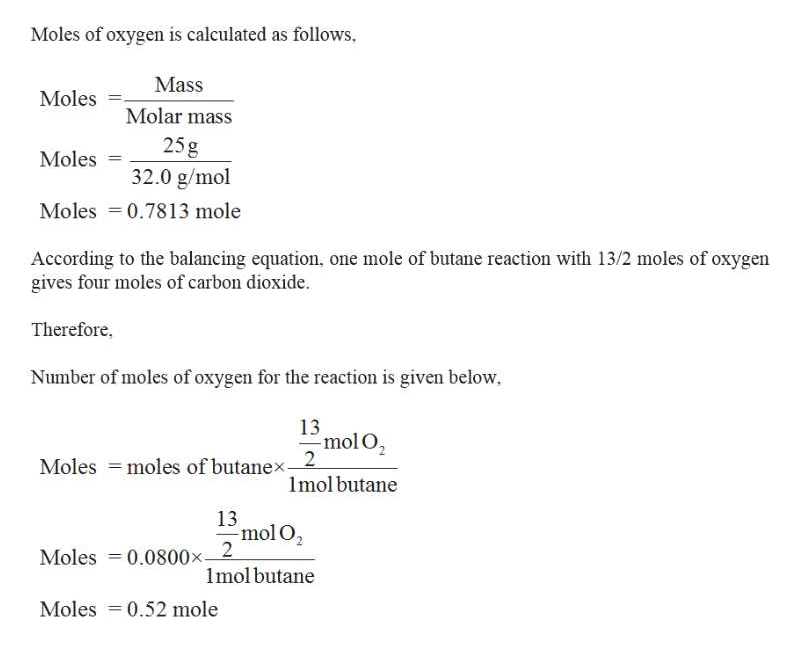# Gaseous butane CH3CH22CH3 will react with gaseous oxygen O2 to produce gaseous carbon dioxide CO2 and gaseous water H2O. Suppose 4.65 g of butane is mixed with 25. g of oxygen. Calculate the maximum mass of carbon dioxide that could be produced by the chemical reaction. Be sure your answer has the correct number of significant digits.

Question
15 views

Gaseous butane

CH3CH22CH3

will react with gaseous oxygen

O2

to produce gaseous carbon dioxide

CO2

and gaseous water

H2O

. Suppose 4.65 g of butane is mixed with 25. g of oxygen. Calculate the maximum mass of carbon dioxide that could be produced by the chemical reaction. Be sure your answer has the correct number of significant digits.

check_circle

Step 1

Given,
4.65 g of butane mixed with 25.0 g of oxygen.help_outlineImage TranscriptioncloseThe balancing equation for the combustion reaction is given below 13 0, (g)4CO, (g)+5H2Og) 2 C,H1 g)+ According to the balancing equation, one mole of butane reaction with 13/2 moles of oxygen gives four moles of carbon dioxide Moles of butane is calculated as follows Mass Moles 11 Molar mass 4.65g 58.12 g/mol Moles Moles 0.0800 mole fullscreen
Step 2

The required O2 is 0.52 mole, but according to the given reaction moles of oxygen is 0.7813 moles. Therefore but...help_outlineImage TranscriptioncloseMoles of oxygen is calculated as follows Mass Moles Molar mass 25g 32.0 g/mol Moles Moles 0.7813 mole According to the balancing equation, one mole of butane reaction with 13/2 moles of oxygen gives four moles of carbon dioxide Therefore Number of moles of oxygen for the reaction is given below 13 mol O2 2 Moles moles of butanex mol butane 13 mol O2 Moles 0.0800x-2 molbutane Moles 0.52 mole fullscreen

### Want to see the full answer?

See Solution

#### Want to see this answer and more?

Solutions are written by subject experts who are available 24/7. Questions are typically answered within 1 hour.*

See Solution
*Response times may vary by subject and question.
Tagged in

### Chemistry